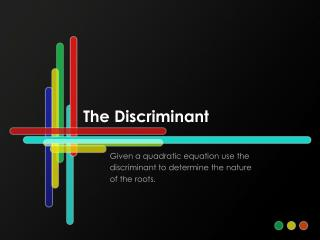DownloadDownload PresentationThe Discriminant

# The Discriminant

Télécharger la présentation## The Discriminant

- - - - - - - - - - - - - - - - - - - - - - - - - - - E N D - - - - - - - - - - - - - - - - - - - - - - - - - - -
##### Presentation Transcript

1. The Discriminant Given a quadratic equation use the discriminant to determine the nature of the roots.

2. What is the discriminant? The discriminant is the expression b2 – 4ac. The value of the discriminant can be used to determine the number and type of roots of a quadratic equation.

3. How have we previously used the discriminant? We used the discriminant to determine whether a quadratic polynomial could be factored. If the value of the discriminant for a quadratic polynomial is a perfect square, the polynomial can be factored.

4. Solve These… • Use the quadratic formula to solve each • of the following equations • x2 – 5x – 14 = 0 • 2x2 + x – 5 = 0 • x2 – 10x + 25 = 0 • 4x2 – 9x + 7 = 0

5. Let’s evaluate the first equation. x2 – 5x – 14 = 0 What number is under the radical when simplified? 81 What are the solutions of the equation? –2 and 7

6. If the value of the discriminant is positive, the equation will have 2 real roots. If the value of the discriminant is a perfect square, the roots will be rational.

7. Let’s look at the second equation. 2x2 + x – 5 = 0 What number is under the radical when simplified? 41 What are the solutions of the equation?

8. If the value of the discriminant is positive, the equation will have 2 real roots. If the value of the discriminant is a NOT perfect square, the roots will be irrational.

9. Now for the third equation. x2 – 10x + 25 = 0 What number is under the radical when simplified? 0 What are the solutions of the equation? 5 (double root)

10. If the value of the discriminant is zero, the equation will have 1 real, root; it will be a double root. If the value of the discriminant is 0, the roots will be rational.

11. Last but not least, the fourth equation. 4x2 – 9x + 7 = 0 What number is under the radical when simplified? –31 What are the solutions of the equation?

12. If the value of the discriminant is negative, the equation will have 2 complex roots; they will be complex conjugates.

13. Let’s put all of that information in a chart.

14. Try These. • For each of the following quadratic equations, • Find the value of the discriminant, and • Describe the number and type of roots. • x2 + 14x + 49 = 0 3. 3x2 + 8x + 11 = 0 • 2. x2 + 5x – 2 = 0 4. x2 + 5x – 24 = 0

15. The Answers • x2 + 14x + 49 = 0 • D = 0 • 1 real, rational root • (double root) • 2. x2 + 5x – 2 = 0 • D = 33 • 2 real, irrational roots • 3. 3x2 + 8x + 11 = 0 • D = –68 • 2 complex roots • (complex conjugates) • 4. x2 + 5x – 24 = 0 • D = 121 • 2 real, rational roots

16. Try These. • The equation 3x2+ bx+ 11=0 has one solution at x=1. What is the other solution? • Find the value of a such that the equation ax2+ 12x + 11 = 0 has exactly one solution. What is that solution? • The equation x2 + 243x – 7839 = 0 has two real solutions (why?). What is the sum of these two solutions? What is the product?